Online interactive resources for the Scottish Maths curriculum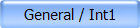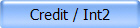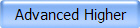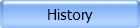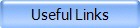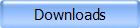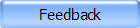Higher Higher Mathematics Resources Worksheets, PowerPoints, Exam Solutions Higher Still Notes - Higher Mathematics (PDF) This website was created by two sixth year students. It contains downloadable PDF notes covering the Higher Maths course. Higher Course Revision Resources (Flash) Downloadable PowerPoint revision aids by topic and notes in PDF. Higher Course Teaching Resources & Interactive Teaching Aids Courtesy of Madras College, Fife. Higher Course Revision by Unit From the McLaren High School Maths Dept. (Select Higher from the home page menu) BBC Bitesize - Higher Revision New in 2003. Good coverage of Higher course with notes, worked examples and on-line tests. BBC Bitesize - AS Level Revision Topics include those applicable to Higher Maths. S-Cool! - A/AS Level Revision Revision topics include those applicable to Higher Maths. A Level Maths Notes (PDF) Well written notes which cover many topics in the Higher Maths course.Gradient Calculation (Shockwave) Determine the gradient from two points. The Straight Line (Java Applet) Graphs a line using either slope and y-intercept, point and slope or two points. Parabolas (Java Applet) Graphs a parabola from coefficients for the equation in both general form, y=ax²+bx+c, and completed square form, y=a(x-h)²+k. Zap It! Game (Shockwave) Try to adjust the quadratic function, y=ax²+bx+c, to 'Zap' as many data points as possible. Unit Circle (Shockwave) Explore the relationship between the unit circle and the graphs of the sine, cosine, and tangent functions. Shifting and Scaling Sine and Cosine Curves (Shockwave) Experiment with the graphs of trigonometric functions of the form y = a sin[b (x - c)] + d. Relate the equation and graph to amplitude, period, and frequency. Logarithms (Shockwave) Experiment with logarithms by changing the base. View the corresponding exponential function as a reflection across the line y = x.Visual Calculus (Java Applet) Links to tutorials & animations (Java & Flash) Calculus 1 (Java Applet) Differentiation Graphical representation of differentiation from first principles. Calculus 2 (Java Applet) Surfing Derivatives Observe the gradient of the tangent as you move (surf) it along the curve. Calculus 3 (Java Applet) Tangent Lines Animation showing the tangent line to a point on a curve, varying as the point moves along the curve. Calculus 4 (Java Applet) Integration Estimation of area under a curve using rectangles or trapezoids. Calculus 5 (Flash) Visualising the Integral Change the width dx of rectangles under the curve and see sums of the rectangles approximate the area. Calculus 6 Step by Step Differentiation & Integration Enter a function and see the step by step solution. Calculus 7 (Flash) Who Doesn't Want To Be A Millionaire Test your knowledge of differentiation & integration. Can include some questions beyond Higher. Calculus 8 (Java Applet) Graphing the Derivative Excellent on-line exercise. Given a function select the graph of its derivative.